# NML:List of tile slopes

Tiles in TTD can have various slopes. The slope is a bitmask that may contain the following bits:

bit flag meaning
CORNER_W west corner is above the lowest corner.
CORNER_S south corner is above the lowest corner.
CORNER_E east corner is above the lowest corner.
CORNER_N north corner is above the lowest corner.
IS_STEEP_SLOPE this tile is a steep slope (the corner opposite to the lowest corner is 2 units higher).

The resulting, possible values of this bitmask are given in the following table. The image below that illustrates them graphically. See also the builtin function `num_corners_raised(slope)`.

Sprites for slopes are always in the same order in TTD. For example, `GROUND_SPRITE_NORMAL` is the sprite number of a flat grass tile. It is followed by 18 other sprites, that display a flat grass tile for each possible slope. The order of these sprites is given in the last column of the table. The builtin function `slope_to_sprite_offset(slope)` can be used to determine the sprite offset of a given slope.

Sprite Named constant Equivalent bitmask Numerical value Sprite offset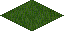SLOPE_FLAT `bitmask()` 0 0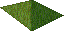SLOPE_W `bitmask(CORNER_W)` 1 1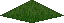SLOPE_S `bitmask(CORNER_S)` 2 2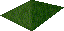SLOPE_E `bitmask(CORNER_E)` 4 4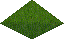SLOPE_N `bitmask(CORNER_N)` 8 8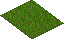SLOPE_NW `bitmask(CORNER_N, CORNER_W)` 9 9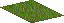SLOPE_SW `bitmask(CORNER_S, CORNER_W)` 3 3SLOPE_SE `bitmask(CORNER_S, CORNER_E)` 6 6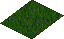SLOPE_NE `bitmask(CORNER_N, CORNER_E)` 12 12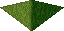SLOPE_EW `bitmask(CORNER_E, CORNER_W)` 5 5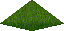SLOPE_NS `bitmask(CORNER_N, CORNER_S)` 10 10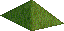SLOPE_NWS `bitmask(CORNER_N, CORNER_W, CORNER_S)` 11 11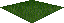SLOPE_WSE `bitmask(CORNER_W, CORNER_S, CORNER_E)` 7 7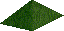SLOPE_SEN `bitmask(CORNER_S, CORNER_E, CORNER_N)` 14 14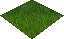SLOPE_ENW `bitmask(CORNER_E, CORNER_N, CORNER_W)` 13 13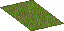SLOPE_STEEP_W `bitmask(CORNER_N, CORNER_W, CORNER_S, IS_STEEP_SLOPE)` 27 17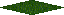SLOPE_STEEP_S `bitmask(CORNER_W, CORNER_S, CORNER_E, IS_STEEP_SLOPE)` 23 16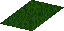SLOPE_STEEP_E `bitmask(CORNER_S, CORNER_E, CORNER_N, IS_STEEP_SLOPE)` 30 18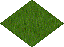SLOPE_STEEP_N `bitmask(CORNER_E, CORNER_N, CORNER_W, IS_STEEP_SLOPE)` 29 15